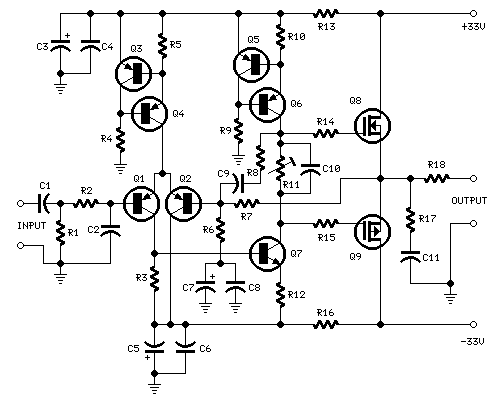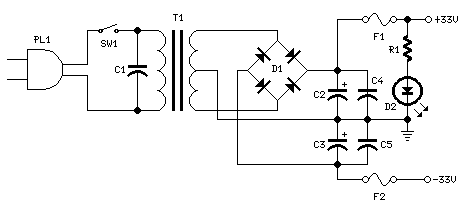# 25W场效应晶体管音频放大器

25W 场效应晶体管（MOSFET）音频放大器电路R1，R4 = 47K 1/4W电阻
R2 = 4K7 1/4W电阻
R3 = 1K5 1/4W电阻
R5 = 390R 1/4W电阻
R6 = 470R 1/4W电阻
R7 = 33K 1/4W电阻
R8 = 150K 1/4W电阻
R9 = 15K 1/4W电阻
R10 = 27R 1/4W电阻
R11 = 500R 1/2W金属陶瓷微调
R12，R13，R16 = 10R 1/4W电阻
R14，R15 = 220R 1/4W电阻
R17 = 8R2 2W电阻
R18 = R22 4W电阻（绕线）
C1 = 470nF的63V涤纶电容器
C2 = 330pF的63V聚苯乙烯电容器
C3，C5 = 470uF的63V电解电容器
C4，C6，C8，C11 = 100nF的63V涤纶电容器
C7 = 25V 100uF的电解电容
C9 = 10pF的63V聚苯乙烯电容器
C10 = 1uF的63V涤纶电容
Q1-Q5 = BC560C 45V100mA低噪音高增益PNP晶体管
Q6 = BD140 80V 1.5A PNP晶体管
Q7 = BD139 80V 1.5A NPN晶体管
Q8 = IRF532 100V 12A N沟道场效应晶体管
Q9 = IRF9532 100V 10A P沟道场效应晶体管R1 = 3K3 1/2W电阻
C1 = 10nF的1000V涤纶电容器
C2，C3 = 4700u901，F 50V电解电容器
C4，C5 = 100nF的63V涤纶电容器
D1 200V 8A二极管
D25毫米。 红色LED
F1，F2 3.15A的保险丝插座
T1 220V，25 + 25V，120VA电源变压器
PL1 主电源插头
SW1单刀单掷（SPST）电源开关

Q6和Q7必须有一个小的U形散热片。
Q8和Q9必须安装在散热片。